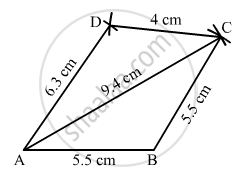Share

# Construct a Quadrilateral Abcd Such that Ab = Bc = 5.5 Cm, Cd = 4 Cm, Da = 6.3 Cm and Ac = 9.4 Cm. Measure Bd. - Mathematics

Course
ConceptConstructing a Quadrilateral When Two Diagonals and Three Sides Are Given

#### Question

Construct a quadrilateral ABCD such that AB = BC = 5.5 cm, CD = 4 cm, DA = 6.3 cm and AC = 9.4 cm. Measure BD.

#### Solution

$\text { Steps of construction: }$

$\text { Step I: Draw AB = 5 . 5 cm }$

$\text { Step II: With B as the centre and radius BC = 5 . 5 cm, draw an arc }.$

$\text { Step III: With A as the centre and radius AC = 9 . 4 cm, draw an arc to intersect the arc drawn in Step II at C }.$

$\text { Step IV: With C as the centre and radius CD = 4 cm, draw an arc } .$

$\text { Step V: With A as the centre and radius AD = 6 . 3 cm, draw an arc to intersect the arc drawn in Step IV at D } .$

$\text { Step VI: Join DA, BC, AC and CD} .$

$\text { The quadrilateral ABCD so obtained is the required quadrilateral } .$Is there an error in this question or solution?

#### APPEARS IN

RD Sharma Solution for Mathematics for Class 8 by R D Sharma (2019-2020 Session) (2017 to Current)
Chapter 18: Practical Geometry (Constructions)
Ex. 18.1 | Q: 2 | Page no. 4
Solution Construct a Quadrilateral Abcd Such that Ab = Bc = 5.5 Cm, Cd = 4 Cm, Da = 6.3 Cm and Ac = 9.4 Cm. Measure Bd. Concept: Constructing a Quadrilateral - When Two Diagonals and Three Sides Are Given.
S